Consumer Research > Categorical Response Analysis > Statistical Details for the Categorical Platform
Publication date: 11/29/2021

# Statistical Details for the Categorical Platform

This section contains statistical details for the Categorical platform.

## Rao-Scott Correction

The Rao-Scott correction is applied to the test of response homogeneity for multiple responses. See Lavassani et al. (2009).

In the case of a multiple response, you can have overlapping samples, meaning a single participant can provide more than one response. The Pearson chi-square test is not appropriate for multiple responses, because the multiple responses violate the Pearson chi-square test assumption of independence. In addition, expected values calculated using the marginal totals are influenced by the multiple responses because the totals are larger than if multiple responses were not allowed.

The Rao-Scott chi-square statistic is defined as follows: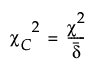where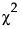is the standard Pearson Chi-squared statistic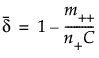is the correction factor

The correction factor contains the following quantities: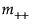is the total count of the multiple responses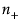is the total number of participants and

C is the number of response levels (number of columns in the Crosstab table).

The degrees of freedom are (R-1)C or the number of rows minus 1 times the number of columns.